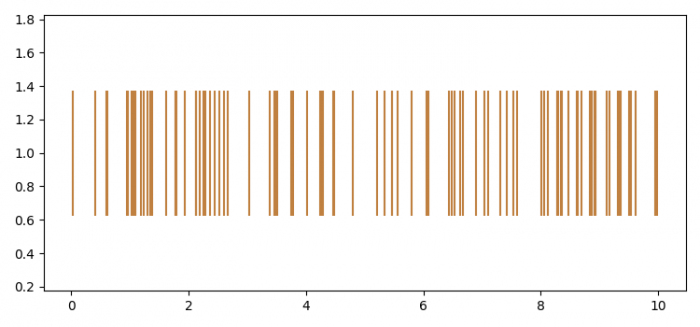# How to pass RGB color values to Python's Matplotlib eventplot?

MatplotlibPythonData Visualization

To pass RGB color values to Python's Matplotlib eventplot, we can take the following steps

## Steps

• Set the figure size and adjust the padding between and around the subplots.
• Create a 1D array, pos, to define the positions of one sequence of events
• Make a list of color tuple r, g, b.
• Plot identical parallel lines at the given positions.
• To display the figure, use show() method.

## Example

import numpy as np
import matplotlib.pyplot as plt

plt.rcParams["figure.figsize"] = [7.50, 3.50]
plt.rcParams["figure.autolayout"] = True

pos = 10 * np.random.random(100)
colors = [(0.75, 0.50, 0.25)]

plt.eventplot(pos, orientation='horizontal',
linelengths=0.75, color=colors)

plt.show()

## OutputYou can change the R, G, B values inside colors to get different colors in the plot.